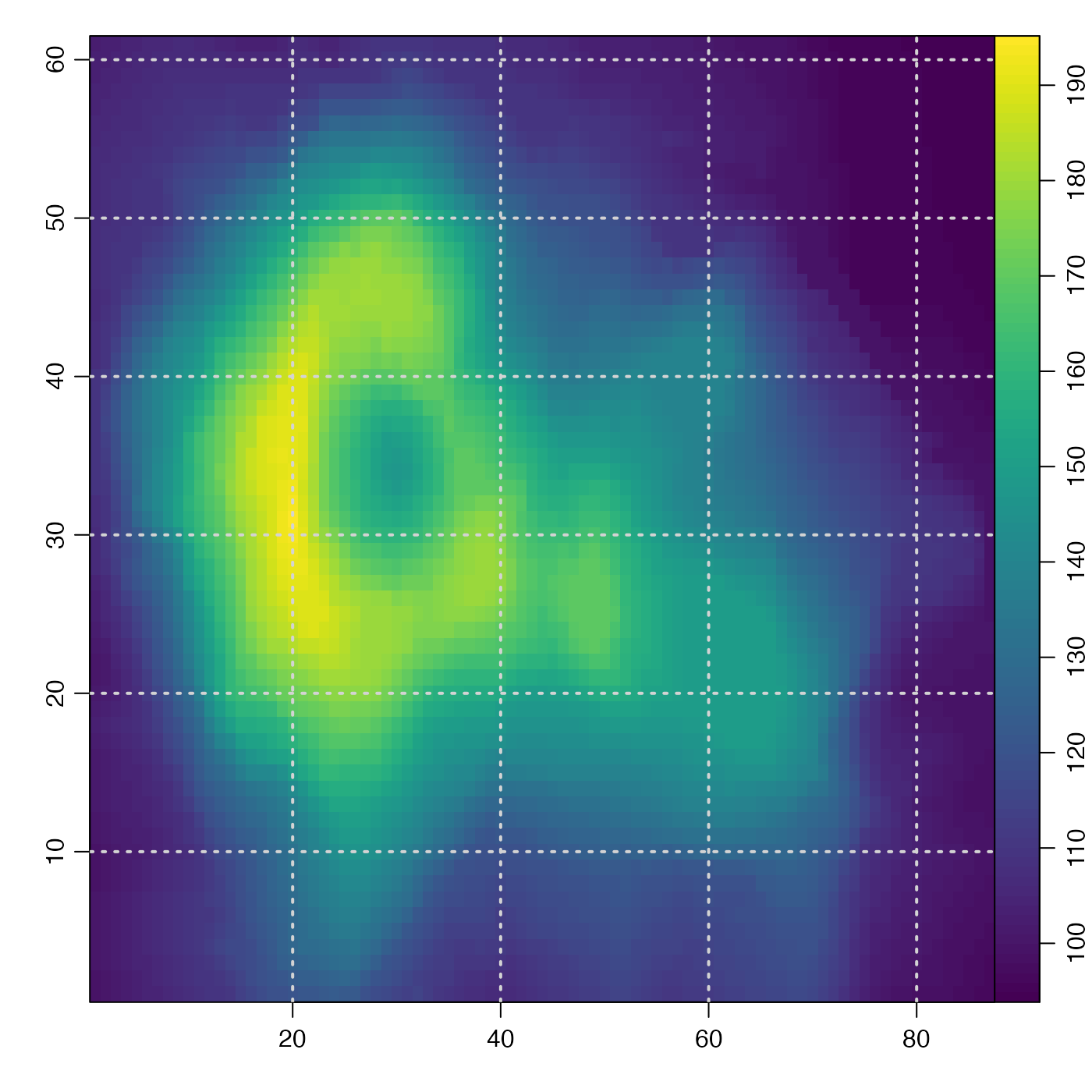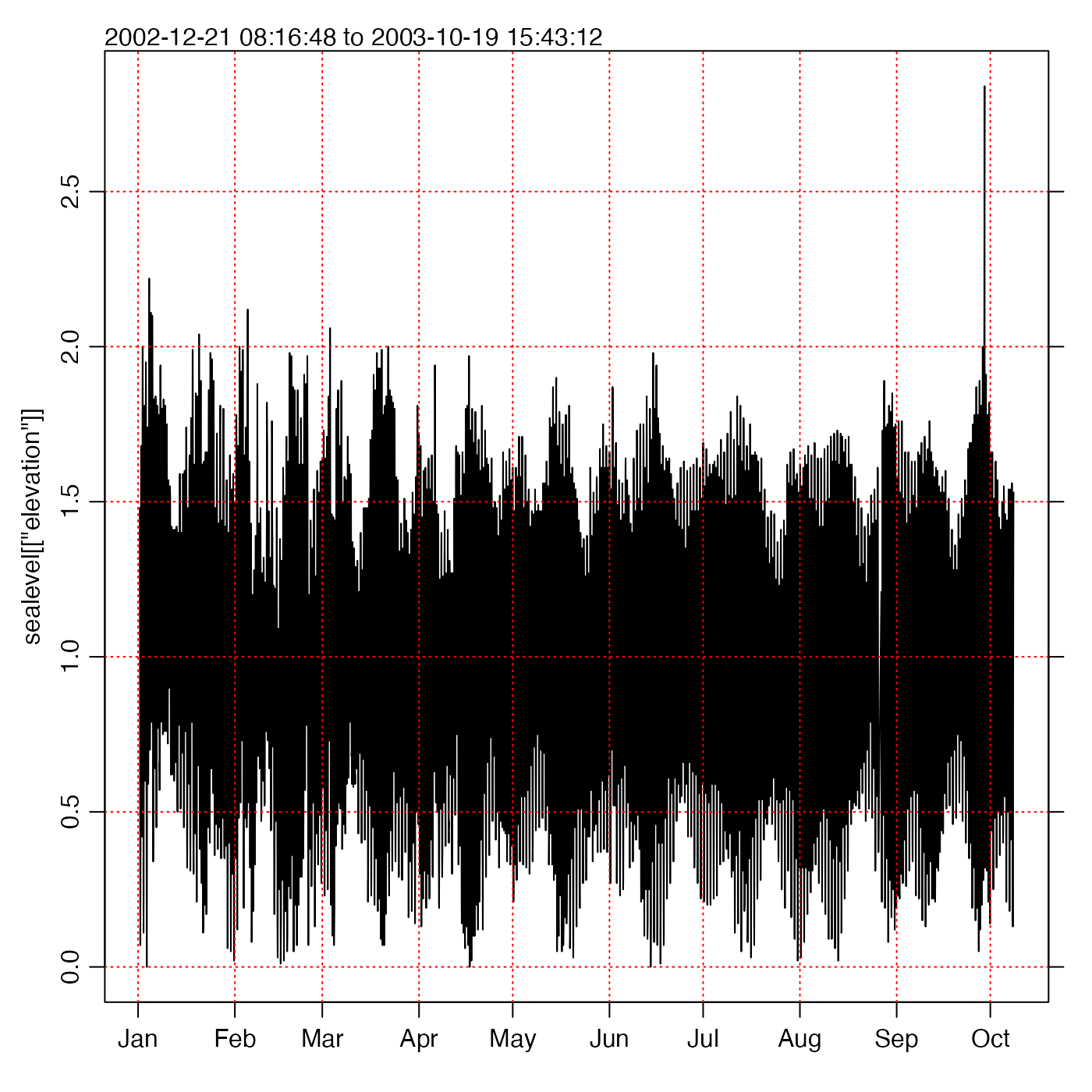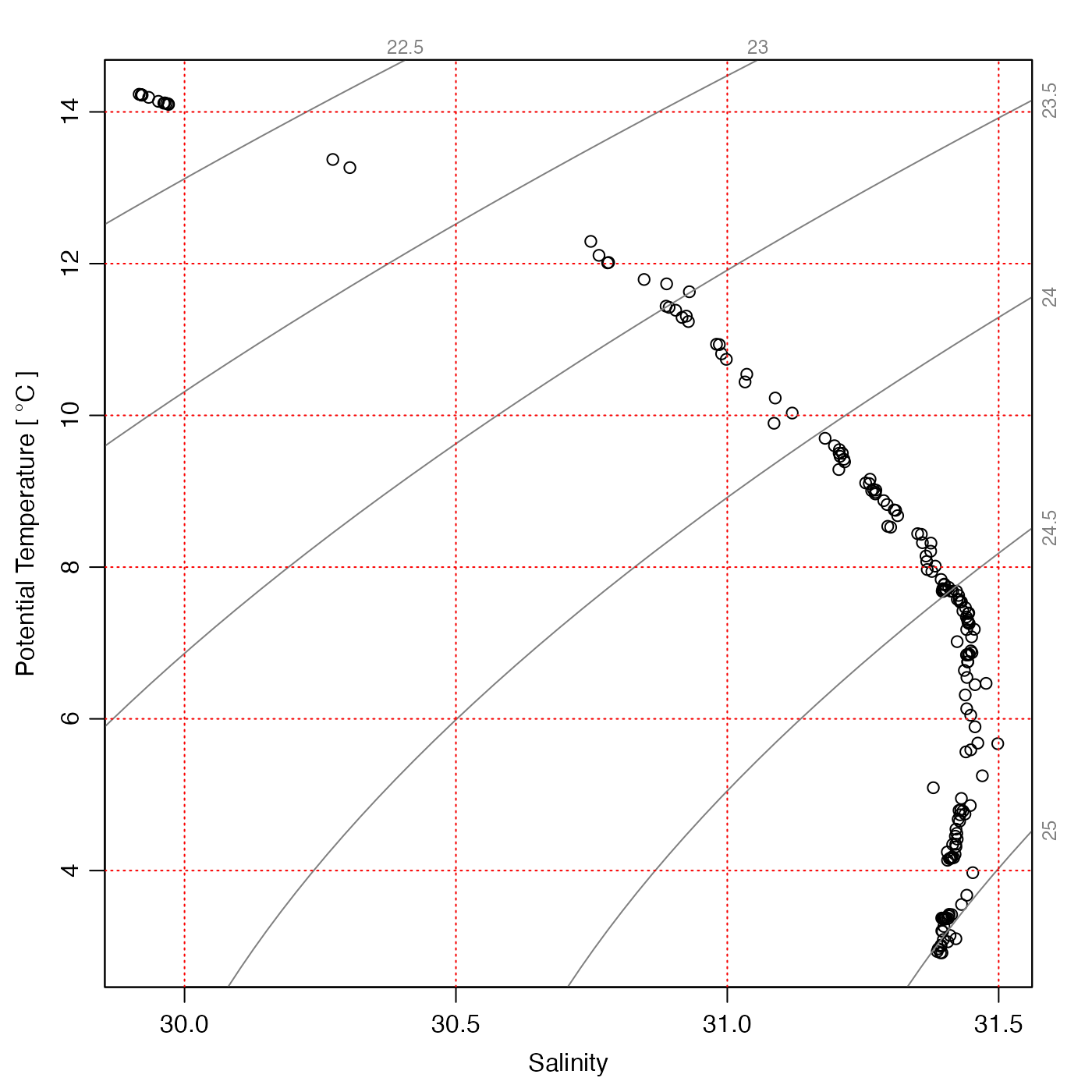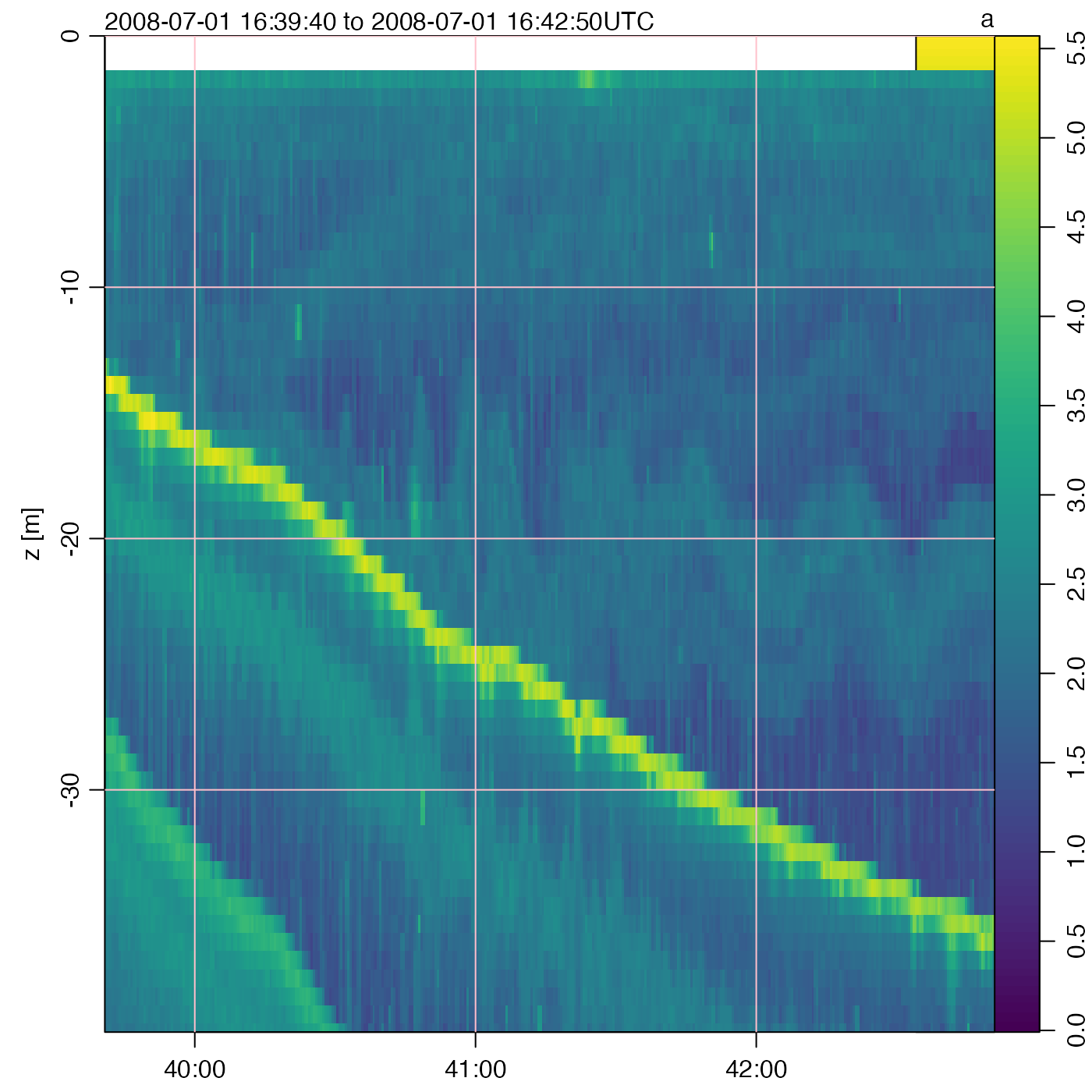Add a Grid to an Existing Oce Plot

oce.grid(xat, yat, col = "lightgray", lty = "dotted", lwd = par("lwd"))

## Arguments

xat either a list of x values at which to draw the grid, or the return value from an oce plotting function a list of y values at which to plot the grid (ignored if gx was a return value from an oce plotting function) color of grid lines (see par()) type for grid lines (see par()) width for grid lines (see par())

## Details

For plots not created by oce functions, or for missing xat and yat, this is the same as a call to grid() with missing nx and ny. However, if xat is the return value from certain oce functions, a more sophisticated grid is constructed. The problem with grid() is that it cannot handle axes with non-uniform grids, e.g. those with time axes that span months of differing lengths.

As of early February 2015, oce.grid handles xat produced as the return value from the following functions: imagep() and oce.plot.ts(), plot,adp-method(), plot,echosounder-method(), and plotTS(). It makes no sense to try to use oce.grid for multipanel oce plots, e.g. the default plot from plot,adp-method().

## Examples

library(oce)
i <- imagep(volcano)oce.grid(i, lwd=2)data(sealevel)
i <- oce.plot.ts(sealevel[["time"]], sealevel[["elevation"]])oce.grid(i, col='red')data(ctd)
i <- plotTS(ctd)oce.grid(i, col='red')i <- plot(adp, which=1)oce.grid(i, col='gray', lty=1)i <- plot(echosounder)oce.grid(i, col='pink', lty=1)